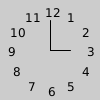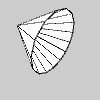# Py5Vector.rotate()#

Rotate vector by a specified angle.

## Examples#```def setup():
py5.translate(py5.width / 2, py5.height / 2)
py5.text_align(py5.CENTER, py5.CENTER)
v = py5.Py5Vector(0, 40)
py5.fill(0)
for i in range(1, 13):
v.rotate(-py5.TWO_PI / 12)
py5.text(f'{i}', v.x, -v.y)
py5.line(0, 0, 0, -30)
py5.line(0, 0, 20, 0)
``````def setup():
py5.size(100, 100, py5.P3D)
py5.translate(10, 20, -20)
# rotate so the viewer can better see the shape
py5.rotate_z(0.5)
py5.rotate_y(-0.5)
v2 = py5.Py5Vector(60, 0, -30)
with py5.begin_shape(py5.TRIANGLE_FAN):
py5.vertex(0, 0, 0)
for i in range(16):
v2.rotate(-py5.TWO_PI / 15, dim='x')
py5.vertex(v2.x, v2.y, v2.z)
```

## Description#

Rotate vector by a specified angle. This method is only applicable to 2D and 3D vectors. Use the `angle` parameter to specify the rotation angle. To rotate 3D vectors, you must use the `dim` parameter to specify which dimension to rotate around. The dimension can be specified with the values 1, 2, or 3, or by using the strings `'x'`, `'y'`, or `'z'`.

A 2D vector will be rotated in the counter-clockwise direction for positive `angle` values and in the clockwise direction for negative `angle` values.

A 3D vector’s rotation will follow the right-hand rule. Using your right hand, point your thumb in the direction of the axis to rotate around. Your fingers will curl in the direction of rotation when the `angle` parameter is positive.

## Signatures#

```rotate(
angle: float,  # angle of rotation, measured in radians
) -> Py5Vector2D

rotate(
angle: float,  # angle of rotation, measured in radians
dim: Union[int, str],  # dimension to rotate around
) -> Py5Vector3D
```

Updated on September 01, 2022 16:36:02pm UTC